Ch 5. Beam Deflections Multimedia Engineering Mechanics Integrationof Moment Integrationof Load Method ofSuperposition IndeterminateBeams
 Chapter 1. Stress/Strain 2. Torsion 3. Beam Shr/Moment 4. Beam Stresses 5. Beam Deflections 6. Beam-Advanced 7. Stress Analysis 8. Strain Analysis 9. Columns Appendix Basic Math Units Basic Equations Sections Material Properties Structural Shapes Beam Equations Search eBooks Dynamics Fluids Math Mechanics Statics Thermodynamics Author(s): Kurt Gramoll ©Kurt GramollMECHANICS - THEORY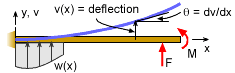Beam Deflection, v, and Slope, dv/dx
Previously, beam stresses and strains were investigated and various equations were developed to predict bending and shear stresses. In addition to stresses, deflection and slope are important and need to be calculated. This section (and this chapter) will deal with various methods to calculate beam deflections.

Deflection Differential Equation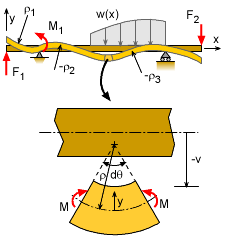Bending Moment, M, and
Radius of Curvature, ρ are related

When a moment acts on a beam, the beam rotates and deflects. The relationship between the radius of curvature, ρ, and the moment, M, at any given point on a beam was developed in the Bending Stress and Strain section as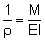This relationship was used to develop the bending stress equation but it can also be used to derive the deflection equation.

Recall from calculus, the radius of curvature for any point of a function, y = f(x), is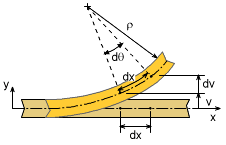Geometry of Beam Bending
and Deflection

For beams, it is convenient to note the deflection as v (upward is positive) instead of y. Also, for small deflections, the first derivative or slope, dy/dx, is small. If it is squared, then it is very small and can be assumed to be zero. In other words, (dv/dx)2 ≈ 0. This simplifies the radius of curvature equation to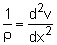Combining this with the bending stress equation gives standard moment-curvature equation,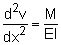This differential equation is also commonly written as

 EIv´´ = M

where the prime, ´ , represents a derivative with respect to x. Since there are two derivatives, there are two slashes. (It is good to recall from dynamics, a dot above a letter represents a time derivative, but a slash represents a spatial derivative.)

Solving the Deflection Differential Equation (Moment-Curvature Equation)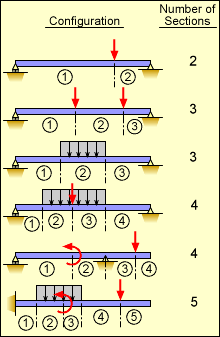Each Beam Section Requires its
Own Deflection Equation

The differential equation EIv´´= M is not useful by itself but needs to be applied to a beam with specific boundary conditions. Generally, EI is constant and M is a function of the beam length. Integrating the equation once gives,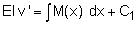Integration again gives,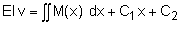The integration constants, C1 and C2, are determined from the boundary conditions. For example, a pinned joint requires the deflection, v, equals 0. A fixed joint requires both the deflection, v, and slope, v´, equal 0. Each beam section must have at least two boundary conditions.

Each beam span must be integrated separately, just like when constructing a moment diagram. If the moment curve is discontinuous, then a single equation cannot model the deflection. Thus, each new support or load will start a new beam section that must be integrated. Examples of beam sections are shown at the left.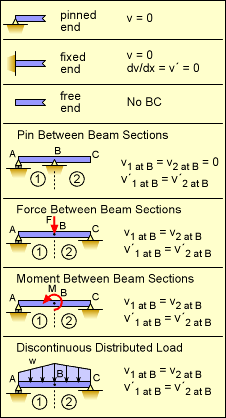Boundary Conditions for Beam Sections
Boundary Conditions

Determining the boundary conditions is usually the most difficult part of solving the deflection differential equation. In particular, boundary conditions for multiple beam sections can be confusing.

For multiple beam sections, many times the boundary between the sections creates a boundary. These type of conditions are also called "Continuity Conditions". For example, a point force on a beam causes the deflections to be split into two equations. However, the beam's deflection and slope will be continuous at the load location requiring v1 = v2 and v´1 = v´2. These conditions are needed to solve for the additional integration constants.

The table at the left summarizes most common boundary (and continuity) conditions for beam deflection and slope.

Practice Homework and Test problems now available in the 'Eng Mechanics' mobile app
Includes over 400 problems with complete detailed solutions.
Available now at the Google Play Store and Apple App Store.